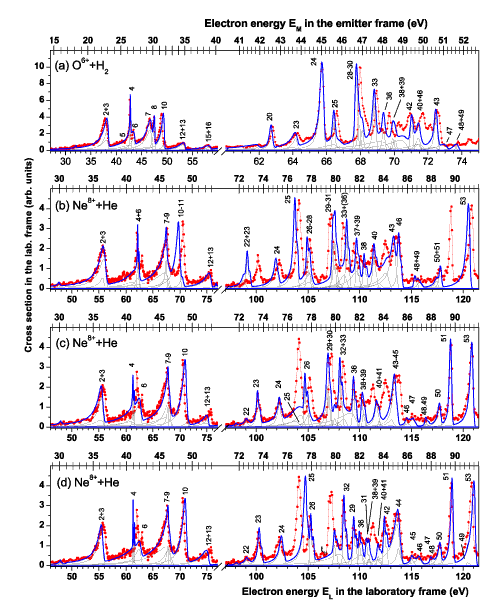Skip Navigation Links.

### Custom Panel Graph with Line + Symbol Plots of Spectroscopic DataComments

The measured electron energy EL is given in the bottom X-axis; the region of interest is selected by using an axis break controlled by the 'axis dialog box' Measured intensities are given in the Y-axis. Opposite X and Y axes are plotted (option in the 'grid lines' tab of the axis dialog box). On the upper non-linear x-scale are added Doppler-free electron energies EM which are related to EL by a nonlinear relation; this new scale, which cannot be produced directly, is created from the following worksheet dataset. EM values are first tabulated with the correct step (scale increment which must appear in the upper X scale); corresponding EL energies are then calculated in a second column with the help of a non-linear relation introduced in the 'set column values dialog box' EL values appearing in the break region of the bottom X axis are eliminated. Corresponding Y values are entered in a third column (in the present case, a constant value corresponding to the left axis maximum Y value). Then, the Y=f(EL) values are added as new data in the layer which are superimposed on the upper X scale; they are displayed as vertical bars with the help of the 'plot details' dialog box (minor ticks in the upper X scale). Having chosen the major tick increment among the EM values, the corresponding EL values (EL) are copied in a fourth column; the Y=f(EL) data are also plotted as vertical bars but with an enlarged size. In the last column are copied the EM(EL) values as labels; size and vertical positions of these labels are controlled with the help of the 'plot details' dialog box. In this way all the EM values (vertical bars on the upper X scale) appear at the correct EL positions (bottom X axis).

Experimental data appear as red (line+point) symbols, theoretical data as gray and blue lines. Each gray line gives the contribution of a single atomic state, blue line is the sum of these individual contributions to be compared with the experimental spectrum. Line intensities of theoretical lines are chosen to illustrate the disagreement between theory and experiment; a true fitting procedure cannot be applied because of too large discrepancies. This adjustment procedure is developed with the help of another application, independent of Origin. All these data are then imported using the import ASCII data possibility of Origin; in the present figure more than two hundred worksheet data are plotted in this way. Plot of the large number of gray lines is controlled with the help of the 'group/ungroup' possibility of the 'layer dialog box' of Origin. Labels on top of some lines are added for the purpose of identification.

 © OriginLab Corporation. All rights reserved.